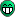# When to call clear() in a vector of vectors

Hi,

I have the following question. Say I am trying to fill a vector<vector > using the following:

``````  TFile *f = new TFile(file_name, "RECREATE");
TTree *tree = new TTree("tree", "tree");
tree->Branch("_parFloatVal","vector<vector<float> >", &_parFloatVal);

std::vector<std::vector<float> > _parFloatVal;
std::vector<float> floatVec;

for (int i = 0; i < 10; i++) {
_parFloatVal.clear();

for (int j = 0; j < 10; j++) {
floatVec.clear();

for (int k = 0; k < 10; k++) {
floatVec.push_back(old_vector->at(j)[k]); // just an example
} // for (int k ...
_parFloatVal.push_back(floatVec);
} // for (int j ...

tree->Fill();
} // for (int i ...

tree->Write();``````

Is the second clear() necessary (floatVec.clear()) in order to avoid pushing back elements to a vector that already has filled elements?

Try without “clearing” it